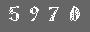# 接口技术

译者: gashero从程序员的角度，端口就像是Erlang进程。你可以发送消息给它，你可以注册(register)它，等等。如果扩展程序crash了，那么连接程序就会收到退出信号，如果连接进程死掉了，扩展进程也会被kill掉。

# 1   端口

`Port=open_port(PortName,PortSettings)`

Port ! {PidC,{command,Data}} ：发送数据到端口

Port ! {PidC,{connect,Pid1}} ：改变控制进程的PID，从PidC到Pid1

Port ! {PidC,close} ：关闭端口

```receive
{Port,{data,Data}} ->
... 数据处理 ...```

# 2   扩展C程序的接口

```int twice(int x) {
return 2*x;
}

int sum(int x, int y) {
return x+y;
}```

```X1=example1:twice(23),
Y1=example1:sum(45,32),```

• 所有包都以2字节的长度代码开头，后面跟着这些字节的数据。
• 想要调用 twice(N) ，Erlang程序必须以特定形式编码函数调用。我们假设编码是2字节序列 [1,N] ；参数1表示调用函数 twice ，后面的N代表一个1字节的参数。
• 调用 sum(N,M) ，我们编码请求到字节序列 [2,N,M] 。
• 假设返回值都是单一的字节长度的。

1. 端口发送字节序列 0,3,2,45,32 到扩展程序。头两个字节0，3，表示包的长度是3，代码2表示调用扩展的 sum 函数，而 45 和 32 表示调用函数的参数。
2. 扩展程序从标准输入(stdin)读取这5个字节，调用 sum 函数，然后把字节序列 0,2,77 写到标准输出(stdout)。前两个字节表示包长度，后面的77是结果(仍然是1字节长度)。

## 2.1   C程序

• example1.c ：这个包含了我们需要调用的原始函数。
• example1_driver.c ：实现了字节流协议和对 example1.c 的调用例程。
• erl_comm.c ：包含读写内存缓冲区的例程。

example1_driver.c

```#include <stdio.h>
typedef unsigned char byte;

int write_cmd(byte* buff, int len);

int main() {
int fn, arg1, arg2, result;
byte buff;

fn=buff;
if (fn==1) {
arg1=buff;
result=twice(arg1);
} else if (fn==2) {
arg1=buff,
arg2=buff,
//如果希望调试，可以写到stderr
//fprintf(stderr,"call sum %i %i\n",arg1,arg2);
result=sum(arg1,arg2);
}
buff=result;
write_cmd(buff,1);
}
}```

erl_comm.c 是对stdin和stdout读写带有2字节包头的数据包的代码。这么写是允许有分片的。

```#include <unistd.h>
typedef unsigned char byte;

int write_cmd(byte* buf, int len);
int write_exact(byte* buf, int len);

int len;
return(-1);
len=(buf<<8) | buf;
}

int write_cmd(byte* buf, int len) {
byte li;
li=(len>>8) & 0xff;
write_exact(&li,1);
li=len & 0xff
write_exact(&li,1);
return write_exact(buf,len);
}

int read_exact(byte* buf, int len) {
int i, got=0;
do {
return i;
got+=i;
} while (got<len);
return len;
}

int write_exact(byte* buf, int len) {
itn i,wrote=0;
do {
if ((i=write(1,buf+wrote,len-wrote)<=0)
return i;
wrote+=i;
} while(wrote<len);
return len;
}```

## 2.2   Erlang程序

Erlang端的驱动程序参考如下:

```-module(example1).
-export([start/0,stop/0]).
-export([twice/1,sum/2]).

start() ->
spawn(fun() ->
register(example1,self()),
process_flag(trap_exit,true),
Port=open_port({spawn,"./example1"},[{packet,2}]),
loop(Port)
end).

stop() ->
example1 ! stop.

twice(X) -> call_port({twice,X}).
sum(X,Y) -> call_port({sum,X,Y}).

call_port(Msg) ->
example1 ! {call,self(),Msg},
{example1,Result} ->
Result
end.

loop(Port) ->
{call,Caller,Msg} ->
Port ! {self(),{command,encode(Msg)}},
{Port,{data,Data}} ->
Caller ! {example1,decode(Data)}
end,
loop(Port);
stop ->
Port ! {self(),close},
{Port,closed} ->
exit(normal)
end;
{'EXIT',Port,Reason} ->
exit({port_terminated,Reason})
end.

encode({twice,X}) -> [1,X];
encode({sum,X,Y}) -> [2,X,Y];

decode([Int]) -> Int.```

`Port=open_port({spawn,"./example1"},[{packet,2}])`

makefile

```.SUFFIXES: .erl .beam .yrl

.erl.beam:
erlc -W \$<

MODS=example1 example1_lid.c

all: \${MODS:%=%.beam} example1 example1_drv.so

example1: example1.c erl_comm.c example1_driver.c
gcc -o example1 example1.c erl_comm.c example1_driver.c

example1_drv.so: example1_lid.c example.c
gcc -o example1_drv.so -fpic -shared example1.c example1_lid.c

clean:
rm example example1_drv.so *.beam```

## 2.3   运行程序

```1> example1:start().
<0.32.0>
2> example1:sum(45,32).
77
4> example1:twice(10).
20
...```

1. 例子程序没有尝试统一Erlang和C程序的整数。这里我们假设整数都是单字节的，并且忽略了精度和符号的问题。在现实的应用中必须注意类型和精度的问题。这是个困难的问题，因为erlang管理着不限制大小的整数，而像C一类的语言却必须管理整数的精度等等。
2. 我们无法在运行扩展程序之前调用对应的erlang的函数(也就是运行 example1:start() 之前不能调用对应函数)。我们更希望可以自动的启动。这在18.7节会详细讲解。

# 3   open_port

@spec open_port(PortName,[Opt]) -> Port

PortName是如下之一：

{spawn,Command}

{fd,In,Out}

Opt是如下之一：

{packet,N}

stream

{line,Max}

{cd,Dir}

{env,Env}

# 4   内联驱动

1. example1_lid.erl ：这个是erlang的服务器。
2. example1.c ：包含我们需要调用的C函数，跟上一节的一样。
3. example1_lid.c ：调用 example1.c 中好函数的函数。

Erlang管理接口的代码如下:

```-module(example1_lid).
-export([start/0,stop/0]).
-export([twice/1,sum/2]).

start() ->
start("example1_drv").

start(SharedLib) ->
ok -> ok;
end,
spawn(fun() -> init(SharedLib) end).

init(SharedLib) ->
register(example1_lid,self()),
Port=open_port({spawn,SharedLib},[]),
loop(Port).

stop() ->
example1_lid ! stop.

twice(X) -> call_port({twice,X}).
sum(X,Y) -> call_port({sum,X,Y}).

call_port(Msg) ->
example1_lid ! {call,self(),Msg},
{example1_lid,Result} ->
Result
end.

loop(Port) ->
{call,Caller,Msg} ->
Port ! {self(),{command,encode(Msg)}},
{Port,{data,Data}} ->
Caller ! {example1_lid,decode(Data)}
end,
loop(Port);
stop ->
Port ! {self(),close},
{Port,closed} ->
exit(normal)
end;
{'EXIT',Port,Reason} ->
io:format("~p~n",[Reason]),
exit(port_terminated)
end.

encode({twice,X}) -> [1,X];
encode({sum,X,Y}) -> [2,X,Y].

decode([Int]) -> Int.```

```// example1_lid.c
#include <stdio.h>
#include "erl_driver.h"

typedef struct {
ErlDrvPort port;
} example_data;

static ErlDrvData example_drv_start(ErlDrvPort port, char* buff) {
example_data* d=(example_data*)driver_alloc(sizeof(example_data));
d->port=port;
return (ErlDrvData)d;
}

static void example_drv_stop(ErlDrvData handle) {
driver_free((char*) handle);
}

static void example_drv_output(ErlDrvData handle, char* buff, int bufflen) {
example_data* d=(example_data*)handle;
char fn=buff, arg=buff, res;
if (fn==1) {
res=twice(arg);
} else if (fn==2) {
res=sum(buff,buff);
}
driver_output(d->port,&res,1);
}

ErlDrvEntry example_driver_entry={
NULL,                   // F_PTR init, N/A
example_drv_start,      // L_PTR start, 在端口打开时调用
example_drv_stop,       // F_PTR stop, 在端口关闭时调用
example_drv_output,     // F_PTR output, 当erlang发送数据到端口时
"example1_drv",         // 驱动的名字
NULL,                   // F_PTR control, port_command回调
NULL,                   // F_PTR timeout, 保留
NULL                    // F_PTR outputv, 保留
};

DRIVER_INIT(example_drv) {  //必须与driver_entry中的名字匹配
return &example_driver_entry;
}```

```1> c(example_lid).
{ok,example1_lid}
2> example1_lid:start().
<0.41.0>
3> example1_lid:twice(50).
100
4> example1_lid:sum(10,20).
30```

# 5   注意

http://www.erlang.org/doc/pdf/erl_interface.pdf

Erl interface (ei) 是一系列的C例程和宏，用于编码和解码Erlang的扩展格式。在Erlang端，一个程序使用 term_to_binary 来将Erlang术语串行化，而在C端ei的例程可以把binary解包。ei也可以用于构造binary，而在Erlang使用 binary_to_term 来解包。

http://www.erlang.org/doc/pdf/ic.pdf

Erlang IDL Compiler (ic) 。这是一个Erlang的OMG IDL编译器实现。

http://www.erlang.org/doc/pdf/jinterface.pdf

Jinterface 是一系列Java到Erlang的接口工具。它提供了Erlang类型到Java类型的完全映射，编码和解码Erlang术语，连接Erlang进程等等。还有一大堆的扩展功能。